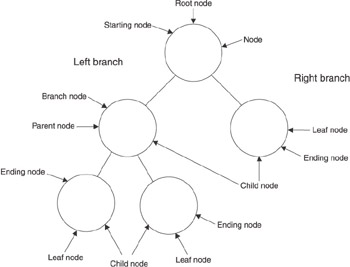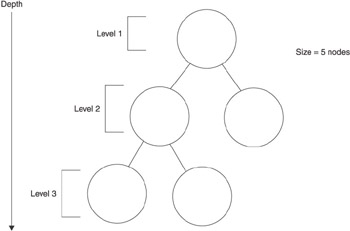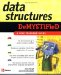# Parts of a Binary Tree

## Parts of a Binary Tree

Although we introduced the concept of a binary tree with terms commonly used when referring to a tree, programmers established different terms to refer to parts of a binary tree. Let s take a moment to become familiar with those terms.

Node is the term used to describe a termination point. There are three kinds of termination points in a binary tree (see Figure 10-2): the starting node, the ending node, and the branch node. The starting node is called the root node , which is the top-level node in the tree. The stem leading from the root node leads to the branch node. The branch node is the fork in the road that links the root node to two branches. Each branch terminates with a child node. Programmers call these the left branch and the right branch .Figure 10-2: A binary tree is comprised of several nodes, each of which are related to other nodes on the tree.

As you can see, a binary tree defines a strong parent-child relationship among nodes. A parent-child relationship is relative to a node. All nodes except the root node have a parent node. However, some nodes have no children, while other nodes have one or two child nodes. Programmers determine the parent-child relationship by selecting a node, which is called the current node. The node that spawns the current node is called the current node s parent node . The node or nodes spawned by the current node is called the child node .

The child node is also referred to as the left node or the right node, depending on which direction the node branches from the current node . If the current node doesn t have any child nodes, then the current node is referred to as the leaf node . A leaf node is located at the bottom of the tree. If you look at your favorite tree, you ll notice that the end of nearly every branch is either another branch (child node) or a leaf, and that s why programmers call a node with no child nodes a leaf node.

### Depth and Size

A binary tree is described by using two measurements: tree depth and tree size (see Figure 10-3). Tree depth is the number of levels in the tree. A new level is created each time a current node branches to a child node. For example, one level is created when the root node branches into child nodes.Figure 10-3: The number of levels in a tree defines a tree s depth, and the number of nodes defines the size of the tree

The size of a tree is the number of nodes in the tree. For example, the first level in Figure 10-3 has one node, which is the root node. The second level has up to two nodes, which are the child nodes of the root. The third level may have up to four nodes. Programmers estimate the size of a tree by using the following formula.

size » 2 depth

Let s say the binary tree has five levels, which is a depth of 5. Here s how you estimate the size of the tree:

32 » 2 5

The size is an approximation because the tree may or may not be balanced. A balanced tree is a binary tree where each current node has two child nodes. An unbalanced tree is a binary tree where one or more current nodes have fewer than two child nodes. This formula gives you a rough idea of how well balanced the tree is. Binary trees are usually used for very large data sets. The formula is not terribly accurate for the small tree shown in Figure 10-3.Data Structures Demystified (Demystified)
ISBN: 0072253592
EAN: 2147483647
Year: 2006
Pages: 90

Similar book on Amazon